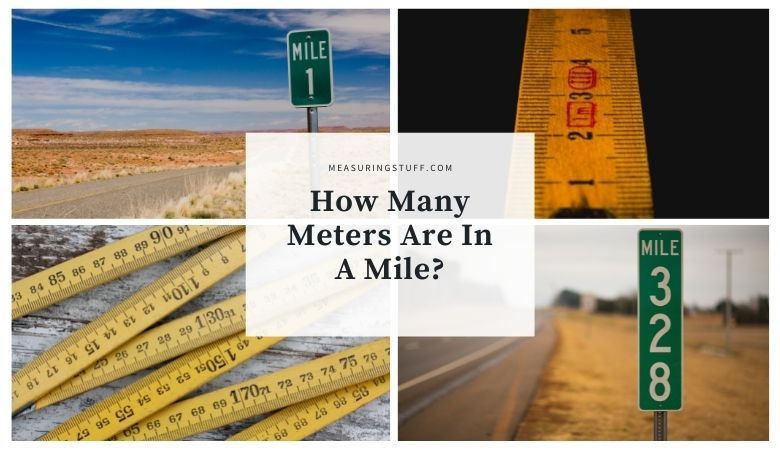# How Many Meters Are In A Mile?

Last updated on September 4th, 2022 at 09:44 pm

Although they’re parts of different measurement systems, meters and miles are both used to measure distance.

Meters are a shorter measurement and are almost the same length as a yard, or 3 feet.

Miles, on the other hand, are a much longer measurement, and they are often used to measure distance traveled by driving.

There are just over 1,609 meters in a single mile.

In order to find out how many meters are in a certain number of miles, you would therefore multiply the number of miles by 1,609.

For example, if you want to know how many meters are in 3 miles, multiply 3 by 1,609.

The answer, which is 4,827, tells you that there are that many meters in 3 miles.

If you want to reverse the question and figure out how many miles can be made from a certain number of meters, you would divide the number of meters by 1,609.

For example, if you have 10,000 meters, you can divide it by 1,609 to get about 6.22.

This means that there are about 6.22 miles in 10,000 meters.

If you would rather avoid doing the math, you can also always use a conversion chart to get a good idea of how many meters there are in a certain number of miles.

## Meters And Miles Measurements

Meters are part of the metric system of measurement, which is common around the world thanks to its ease of use.

CHECK OUT  How Many Acres Is A City Block?

Although the metric system isn’t standard in the United States, it’s still commonly used for scientific measurements.

Miles are part of the imperial system of measurement, which is standard in the United States.

## Meters To Miles Conversion Calculator

Use the following calculator to easily convert meters into yards.